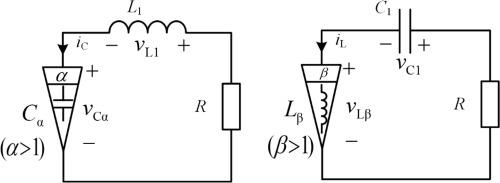Journal of Advanced Research ( IF 5.045 ) Pub Date : 2020-05-22 , DOI: 10.1016/j.jare.2020.05.005
Yanwei Jiang; Bo Zhang; Xujian Shu; Zhihao WeiFractional-order circuit is a kind of circuit which contains fractional-order elements. It has been proved that the fractional-order circuit has some characteristics which are hard to be achieved by integer-order circuits, such as higher degree of freedom in circuit design. For integer-order circuits, there are not only non-autonomous circuits, but also autonomous circuits. Since there are many applications of integral-order autonomous circuits in real world, it is also necessary to explore fractional-order autonomous circuits. However, few research focuses on fractional-order autonomous circuits. Therefore, this paper proposes two kinds of fractional-order autonomous circuits based on fractional-order elements with order larger than one. The corresponding mathematical models are also established based on fractional calculus and their characteristics are analyzed based on circuit theory. Finally, circuit simulation are performed to verify the correctness of theoretical analysis.

down
wechat
bug